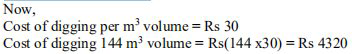# NCERT Solutions Class 9 Mathematics Solutions for Surface Areas and Volumes - Exercise 13.4 in Chapter 13 - Surface Areas and Volumes

Find the cost of digging a cuboidal pit 8 m long, 6 m broad, and 3 m deep at the rate of Rs 30 per \mathbf{m}^{3}

The given pit has its length (l) as 8 m, width (b)as 6 m, and depth (h) as 3 m.

Volume of cuboidal pit = l x b x h = (8 x 6 x 3) = 144 (using formula)

The required Volume is 144 \mathbf{m}^{3}Video transcript
"hello students welcome to lido q a video session i'm saf your math tutor and question for today is find the cost of digging a cuboidal pit 8 meter long 6 meter broad and 3 meter deep at the rate of rupees 30 per meter cube so the information which is given in the questions are length breadth and depth depth is considered as height over here so the dimensions of the cuboidal fit we will write down first length is 8 meter breath that is b that is six meter and depth let us say it as h that is three meter now volume of the cuboidal pit is length into breath into height so this is 8 into 6 into 3 and that is 144 144 meter cube so the required volume is 144 meter cube now it is given that the cost cost is rupees 30 per meter the digging cost is rupees 30 for 1 meter so 144 meter cube cost will be how much that we need to find that will be 144 multiplied by 30 so that will be equal to 4320 rupees so cost of digging 144 meter cube is rupees four three two zero that is our final answer if you have any query regarding this you can drop it in our comment section and subscribe to lido for more such q a thank you for watching"
Related Questions

Lido

Courses

Teachers

Book a Demo with us

Syllabus

Maths
CBSE
Maths
ICSE
Science
CBSE

Science
ICSE
English
CBSE
English
ICSE
Coding

Terms & Policies

Selina Question Bank

Maths
Physics
Biology

Allied Question Bank

Chemistry
Connect with us on social media!